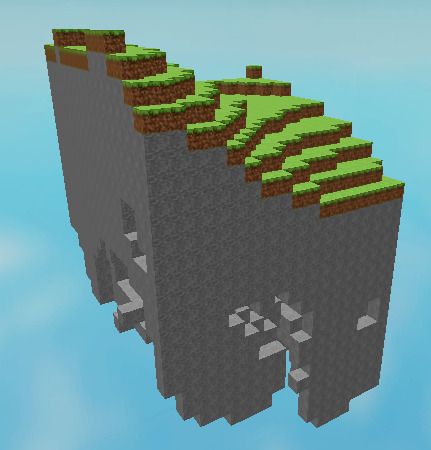# Help with cave system!

Hey there, I am currently working on a Perlin Noise terrain generation just like a game Minecraft. I’ve came pretty close to it but I want to add in a caves system. I heard the best way is to use Perlin Worms. Problem is I have no idea how to use them, even then turning them into a cave route/system. If you have any idea how I can go about this please let me know. Thanks in advance!

PICTURE:CODE SNIPPET:

``````local NoiseScale = 5
local Amplitude = 10

local xNoise = math.noise(y/NoiseScale, z/NoiseScale, Seed) * Amplitude
local yNoise = math.noise(x/NoiseScale, z/NoiseScale, Seed) * Amplitude
local zNoise = math.noise(x/NoiseScale, y/NoiseScale, Seed) * Amplitude
local density = xNoise + yNoise + zNoise + y

local noise1 = math.noise(Seed, (ChunkSize.X/12 * 0) + x/12, (ChunkSize.Z/12 * 0) + z/12) * 8
local noise2 = math.noise(Seed + 10, (ChunkSize.X/36 * 0) + x/36, (ChunkSize.Z/36 * 0) + z/36) * 32
local noise = math.floor((noise1 + noise2) / 2)
local yPos = math.floor(noise) * BlockSize.Y - y * BlockSize.Y
local position = -Vector3.new(0 * ChunkSize.X * BlockSize.X + x * BlockSize.X, yPos, 0 * ChunkSize.Z * BlockSize.Z + z * BlockSize.Z)

if (density > 1) then
local block
if (y == ChunkSize.Y) then
block = Blocks["Grass"]:Clone() block.Size = BlockSize block.Name = block.Name.."Layer("..x..","..z..")" block.Anchored = true
else
block = Blocks["Stone"]:Clone() block.Size = BlockSize block.Name = block.Name.."Layer("..x..","..z..")" block.Anchored = true
end
block.Position = position
block.Parent = chunkFolder
end``````
2 Likes